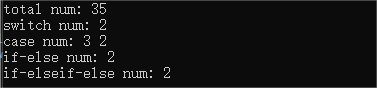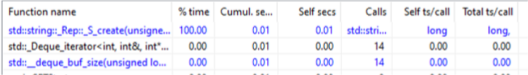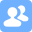# EE308_Lab1-2

 The Link Your Class https://bbs.csdn.net/forums/MUEE308FZU202201 The Link of Requirement of This Assignment https://bbs.csdn.net/topics/608734907 The Aim of This Assignment Programming&Keywords Capturing MU STU ID and FZU STU ID 832001101_20122225
 Personal Software Process Stages Estimated Time/minutes Completed Time/minutes Planning 30 30 Estimate 20 30 Development 30 30 Analysis 50 60 Design Spec 60 70 Design Review 30 30 Coding Standard 30 30 Design 30 30 Coding 60 70 Code Review Planning 40 40 Test 40 30 Reporting 40 50 Test Report 20 30 Size Measurement 30 40 Postmortem & Process Improvement 30 40 total 540 610

3. Ideas to slove the Lab

1. Import the file and save each line of sample code to the array for future search and comparison.

2. Store all keyword categories in an array

3. Compare the sample code array with the keyword group to count the number of code keywords.

4. Understand the relationship between switches and cases, and correctly count the number of switches and cases in each segment

5. Find out the relationship between if, else if and else, design an appropriate algorithm, and calculate the quantity correctly.

4. Design and implementation process

``````#include <string>
#include <iostream>
#include <fstream>
using namespace std;
the_keyword = ["auto", "break", "case", "char", "levelst", "leveltinue", "default", "do",
"double", "else", "enum", "extern", "float", "for", "goto", "if",
"int", "long", "register", "return", "short", "signed", "sizeof", "stastic",
"struct", "switch", "typedef", "union", "unsigned", "void", "else-if", "while"]
f = open(path, "r")``````

Key Code Explanation

1.Establish the variables to store value:

``````now_line = f.readline()
now_line = now_line[:-1]
the_keywords_length = 32
num_a = 0
num_b = []
flag_if = 0
flag_else_if = 0
num_if_else = 0
num_if_else_if_else = 0
sentences = []
x_len = 100000
count = np.zeros(the_keywords_length, dtype=int)
``````

2.Code for level 1 and level 2:

``````while now_line:
now_line = now_line[:-1]
now_line = now_line.replace("else if", "else-if")   ## it's convenient for searching
x = re.split(' |\(|\;|\\t|\:|\{|\/\/', now_line)##split the words from  sentences

for i in x:
if (i == "if" or i == "else" or i == "else-if"):
sentences.append(x)
continue
for i in x:
for j in range(32):
if (i == the_keyword[j]):
if (i == "switch"):
num_b.append(num_a)
num_a = 0
if (i == "case"):
num_a += 1
count[j] += 1

f.close()##close the file
num_b.append(num_a)
sum = 0
for i in range(32):
if (count[i] != 0):
sum += count[i]
if (i == 30):
sum += count[i]``````

3.Code for level 3 and level 4:

``````for i in sentences:
if (len(i) < x_len):
x_len = len(i)

for j in range(x_len):
for i in sentences:
if (i[j] == "if"):
flag_if = 1
if (i[j] == "else-if"):
flag_else_if = 1
if (i[j] == "else"):
if (flag_else_if == 1):
num_if_else_if_else += 1
elif (flag_if == 1):
num_if_else += 1
flag_else_if = 0
flag_else = 0``````

4.Print the result:

``````    print("sum : ", sum)  # 1
print(the_keyword, " num : ", count)  # 2
print("case num", num_b[1:3])  # 2
print("if-else num:", num_if_else)   # 3
print("if-elseif-else num:", num_if_else_if_else)   # 4``````

5.Unit test screenshots and description6.Unit test coverage optimization and performance testing7.Summarize this assignment.

This experiment was my first introduction to Github. I don't know anything about many of the features. There are some difficulties in the specific operation. So, I searched a lot of information and spent a lot of time.
I need to improve my ability to combine theory with practice. I hope to make more progress in the practical operation in the future.

...全文
85 回复 打赏 收藏 转发到动态 举报05 group - topic selection and requirement analysis report
This is the blog of Group 05 for Lab3-1 (Final version).MU-SE: Course summary
Summary Blog for EE308FZ.

285发帖与我相关我的任务• 近7日
• 近30日
• 至今